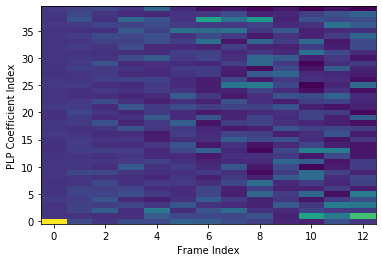# spafe.features.rplp¶

spafe.features.rplp.plp(sig, fs, num_ceps=13, pre_emph=0, pre_emph_coeff=0.97, win_len=0.025, win_hop=0.01, modelorder=13, normalize=0)[source]

compute plps.

Parameters: sig (array) – a mono audio signal (Nx1) from which to compute features. fs (int) – the sampling frequency of the signal we are working with. Default is 16000. num_ceps (float) – number of cepstra to return. Default is 13. pre_emph (int) – apply pre-emphasis if 1. Default is 1. pre_emph_coeff (float) – apply pre-emphasis filter [1 -pre_emph] (0 = none). Default is 0.97. win_len (float) – window length in sec. Default is 0.025. win_hop (float) – step between successive windows in sec. Default is 0.01. win_type (float) – window type to apply for the windowing. Default is “hamming”. modelorder (int) – order of the model / number of cepstra. 0 -> no PLP. Default is 13. normalize (int) – if True apply normalization. Default is 0. plps.
spafe.features.rplp.rastaplp(x, fs=16000, win_len=0.025, win_hop=0.01, do_rasta=True, modelorder=13, normalize=0)[source]

compute rasta Perceptual Linear Prediction coefficients (rasta plp). [cepstra, spectra, lpcas] = rastaplp(samples, sr, do_rasta, modelorder)

Parameters: x (array) – signal array. fs (int) – sampling rate. Default is 1600. win_len (float) – length of window. Default is 0.025, win_hop (float) – window hop. Default is 0.010, do_rasta (bool) – if True apply rasta filtering. If False PLP is calculated. Default is True, modelorder (int) – order of the model / number of cepstra. 0 -> no PLP. Default is 13, normalize (int) – if True apply normalization. Default is 0 PLP or RPLP coefficients. Matrix of features, row = feature, col = frame.
spafe.features.rplp.rplp(sig, fs, num_ceps=13, pre_emph=0, pre_emph_coeff=0.97, win_len=0.025, win_hop=0.01, normalize=0)[source]

compute rasta plps.

Parameters: sig (array) – a mono audio signal (Nx1) from which to compute features. fs (int) – the sampling frequency of the signal we are working with. Default is 16000. num_ceps (float) – number of cepstra to return. Default is 13. pre_emph (int) – apply pre-emphasis if 1. Default is 1. pre_emph_coeff (float) – apply pre-emphasis filter [1 -pre_emph] (0 = none). Default is 0.97. win_len (float) – window length in sec. Default is 0.025. win_hop (float) – step between successive windows in sec. Default is 0.01. normalize (int) – if True apply normalization. Default is 0. rasta plps.

Example:

import scipy.io.wavfile
import spafe.utils.vis as vis
from spafe.features.mfcc import plp